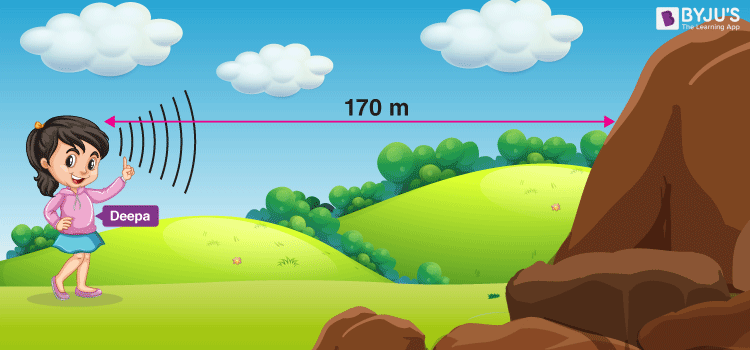# Wave Speed

Let’s assume that you move one end of the rope up and down to generate a wave in the string. How long will it take for the wave to travel from one end to another? It depends on the speed of the wave.

## What is the Speed of a Wave?

Wave speed is the distance the wave travels in a given amount of time, such as the number of meters it travels per second. The equation that represents the wave speed is given as follows:

$$\begin{array}{l}Speed=\frac{Distance}{Time}\end{array}$$
If an ocean wave crest travels a distance of 30 m in 10 seconds, then the speed of the ocean wave is 3 m/s. On the other hand, if the crest of the ocean wave covers a distance of 40 m at the same time that is 10 seconds, then the speed of this ocean wave is 4 m/s. From this, we can conclude that the faster wave covers a larger distance in the same amount of time.

Sometimes, we encounter situations in which the wave meets the end of a medium and also the presence of a different medium. To better understand this, let us take the example of a slinky. The wave introduced by a person into the slinky will travel through the slinky and eventually reaches the end of the slinky and the presence of the hand of a second person. One behavior that waves undergo at the end of the medium is reflection. When a wave undergoes reflection, it merely remains in the medium and only reverses its direction of travel. The slinky wave has traveled double its distance. This reflection phenomenon of waves is commonly observed in sound waves. When you let out a loud cry within a canyon, you often hear the echo of the holler. The sound waves travel through the medium; in this case, the air and reflect off the canyon wall and return to the origin of the sound (you). The result is that you hear the echo of your holler. A classic problem about the reflection of the wave is as follows:

Example 1: Deepa stands 170 meters away from a steep canyon wall. She shouts and hears her echo a second later. What is the speed of the wave?Solution:

In this case, the speed of the wave is 340 m/s because the distance travelled by the sound wave in 1 second is equivalent to 170 meters down to the canyon wall plus 170 meters back from the canyon wall.

And, we know that,

$$\begin{array}{l}Speed=\frac{Distance}{Time}\end{array}$$

Substituting the value, we get:

$$\begin{array}{l}Speed=\frac{170\,m/s + 170\,m/s}{1\,s}\end{array}$$
$$\begin{array}{l}= 340\,m/s\end{array}$$

### Dependance on the Medium

The speed of most waves depends on the medium in which it travels. Generally, waves travel fastest through solids and slowest through gases. This is because particles are closest together in solids and farthest apart in gases. When the particles are farther apart, it takes longer for the energy distribution to pass from one particle to another through the medium.

The given video demonstrates the velocity of the wave on a string.If you wish to learn more Physics concepts with the help of interactive video lessons, download BYJU’S – The Learning App.

## Frequently Asked Questions – FAQs

### What is a wave?

A wave is a disturbance in a medium that carries energy without a net movement of particles.

True.

### Define wave speed.

Wave speed is defined as the total distance covered by the wave in a given time period.

### Give the equation that represents the wave speed.

Wave Speed = Distance Covered/Time taken

### Define period of a wave.

The period of a wave is the time for a particle on a medium to make one complete vibrational cycle.
Test Your Knowledge On Wave Speed!